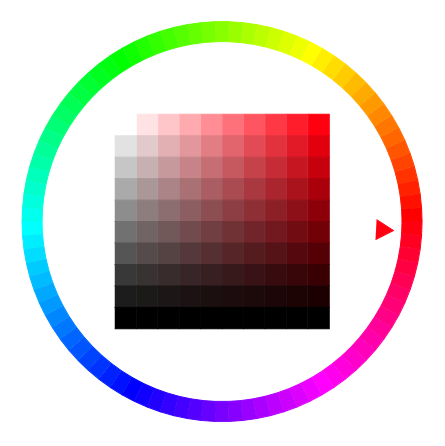### Color class

The easiest way is to use one of the predefined colors. For example:

``````seymour.Color = Colors.Green;
``````

A color is defined based on the RGB color model by its red, green and blue values. If you want to create a color based on its red, green and blue values, it works like this:

``````seymour.Color = new Color(redValue, greenValue, blueValue);
``````

The values for `redValue`, `greenValue` and `blueValue` must be between 0 and 255.

Sometimes you want a shape to be translucent so that what is behind a shape can still be seen. In this case, you can create a transparent color. This can be achieved like this:

``````seymour.Color = Colors.Green.Transparent(0.5);
``````

In this example, a semi-transparent green is created. The value for transparency is called alpha channel, its value can be between 0.0 (fully opaque) and 1.0 (fully transparent). Any color can be created as follows:

``````seymour.Color = new Color(redValue, greenValue, blueValue, alphaChannelValue);
``````

So actually quite simple. You can have any color you want using the correct three values for red, green and blue. Then a quick question: Which r, g, b values do you have to specify if you want the color yellow? You have to specify r = 255 and g = 255 - this is neither plausible nor simple. In reality, color perception is very complex and r, g, b values are not always the best model. Alternatively, colors can also be specified using the HSL and HSV color model. Here, too, you specify three values:

• Hue: Angle on the color circle. The values from 0 to 240 represent the colors of the rainbow: from 0 red to 120 green and 240 blue. The values between 240 and 360 represent the colors on the so-called purple line.
• Saturation: 0.0 neutral gray, 0.5 little saturation, 1.0 pure color.
• Value: 0.0 no brightness (black), 1.0 full brighntess.

In the following example, the outer circle shows all colors with maximum Saturation and Value. In the east (Hue = 0) is red, following the circle on the left are the rainbow colors up to blue (Hue = 240), then the colors of the purple line. The color square in the middle shows the color red for different values of Saturation and Value:To set an HSV color, the `FromHSV` method is used:

``````var newColor = Color.FromHSV(hue, saturation, value);
``````

To determine the HSV values of a color, the `ToHSV` method is used:

``````var red = Colors.Red;
var (hue, saturation, value) = red.ToHSV();
``````

You can also “rotate” a color with a give hue delta:

``````var red = Colors.Red;
var newColor = red.RotateHue(120);
// The new color is a shade of green
``````

If you want to try some things with colors, you can use the examples from `Woopec.Core.Examples.ColorDemo`. The image above is created by this example:

``````public static void WoopecMain()
{
Woopec.Core.Examples.ColorDemo.ColorAnimation();
}
``````

### Speed class

The easiest way is to use a predefined speed:

Speed Description
Speeds.Slowest 100 pixels in one second. Full rotation in 2.3 seconds.
Speeds.Slow
Speeds.Normal
Speeds.Fast 100 pixels in 0.04 seconds. Full rotation in 0.23 seconds.
Speeds.Fastest Fastest speed, no animation

You can also specify Speed using any double value. The value 1 corresponds to Speeds.Slowest, the value 10 corresponds to Speeds.Fast, but higher values can also be used.

There are also a few auxiliary methods:

Method Description
MillisecondsForMovement(Vec2D fromPoint, Vec2D toPoint) Determines for the current speed how many milliseconds are needed to move from one point to another.
MillisecondsForRotation(double oldHeading, double newHeading) Determines for the current speed how many milliseconds are needed for a rotation from one to another angle.

### Vec2D class

A two-dimensional vector class.

Here are a few examples of how to create vectors and lists of vectors:

``````// Create a vector with new:
var v = new Vec2D(3, 4);
// A different way to create a vector:
Vec2D v = (3, 4);
// Create a list of vectors:
var list = new List<Vec2D> { (1, 2), (3, 5) };
// A different way to create a list of vectors (e.g. useful, if you want to pass it to a method)
AMethod(new() { (1, 2), (3, 5) });
``````

The following methods are available:

Method Description
HeadingTo(Vec2D position) Calculate the angle from the end-point of the vector to the given `position`. This angle is given in degrees, such that it can be used as a `Heading` of a `Turtle`